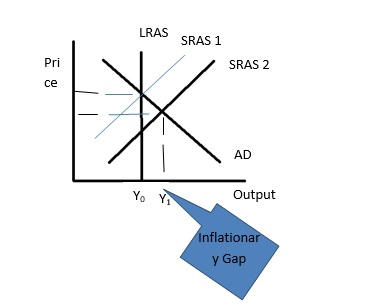# Inflationary Gap

Posted in Finance, Accounting and Economics Terms, Total Reads: 1293

## Definition: Inflationary Gap

Inflationary Gap is the difference between actual GDP and the GDP that can be if there is full in a country. If the difference is positive the gap is represented as an Inflationary Gap and if the difference is negative the gap is represented as a Recessionary Gap.

Inflationary Gap is a term used in Macroeconomics that determines the gap in the GDP which occurs due to the unemployment in a country. The full employment in a country is not possible due to various types of unemployment i.e. cyclical (due to economy), structural (due to skill mismatch) or frictional unemployment (due to switches in jobs). Due to theses unemployment factors the Output gap occurs in the GDP, which is the difference between the actual GDP and the potential GDP. An Output Gap can be described in two ways depending upon whether the actual GDP is more or the potential GDP.

If Potential GDP is more, the gap is referred to as the recessionary gap, while if the Actual GDP is more, the gap is referred to as an inflationary gap. The actual GDP output becomes more than the potential GDP output, due to the inflation onset which increases the prices. First the demand in the country increases, which increases the prices of the goods and service, which in turn increases the real GDP of the country. Hence the gap is created between the real GDP and the potential GDP. The Long run aggregate supply (LRAS) curve is vertical due to the fact that changes in demand (aggregate demand) is only temporary. And short run aggregate supply (SRAS) curve is shifted towards right due to the increase in demand.Using the above figure, formula for inflationary gap can be recognized as below:

Inflationary Gap = GDPactual(Y1) - GDPpotential(Y0)

For Example: Suppose there is 80 percent people employed in a country A. Let the GDP at this level of employment for the country A be GDP’. Now let us consider that GDP at full level of employment in the country A is GDP’’. Therefore the difference in the two GDPs i.e. (GDP’’-GDP’) will be referred to as inflationary gap in the country A.

Hence, this concludes the definition of Inflationary Gap along with its overview.

Browse the definition and meaning of more terms similar to Inflationary Gap. The Management Dictionary covers over 7000 business concepts from 6 categories. This definition and concept has been researched & authored by our Business Concepts Team members.

Search & Explore : Management Dictionary

Similar Definitions from same Category: